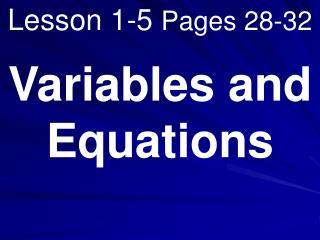DownloadDownload PresentationLesson 1-5 Pages 28-32

# Lesson 1-5 Pages 28-32

Télécharger la présentation## Lesson 1-5 Pages 28-32

- - - - - - - - - - - - - - - - - - - - - - - - - - - E N D - - - - - - - - - - - - - - - - - - - - - - - - - - -
##### Presentation Transcript

1. Lesson 1-5 Pages 28-32 Variables and Equations

2. What you will learn! • How to identify and solve open sentences. • How to translate verbal sentences into equations.

3. Vocabulary

4. What you really need to know! An equation that contains a variable is called an open sentence. A value that makes the sentence true is called a solution.

5. Properties of Equality:

6. Balance Example!

7. Example 1: Find the solution of: 44 + p = 53. Is it 11, 9, or 7? p is 9 ; p = 9

8. Example 2: Which value is the solution of 4x – 1 = 11? A 5 B 4 C 3 D 2 C 3

9. Example 3: Solve the equation mentally. 7x = 56 x = 8

10. Example 4: Solve the equation mentally. x – 15 = 40 x = 55

11. Example 5: Name the property shown by the statement. If 3x + 1 = 10, then 10 = 3x + 1. Symmetric

12. Example 6: Name the property shown by the statement. If z + 6 = 8 and 8 = 2 + 6, the z + 6 = 2 + 6. Transitive

13. Example 7: Define a variable. Then write an equation and solve. The quotient of a number and four is nine. n ÷ 4 = 9 n = 36